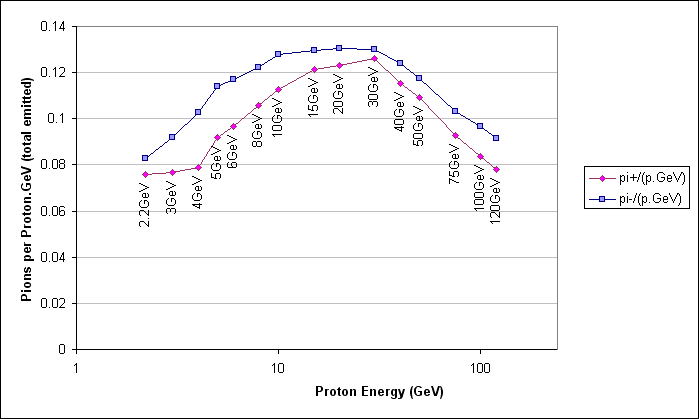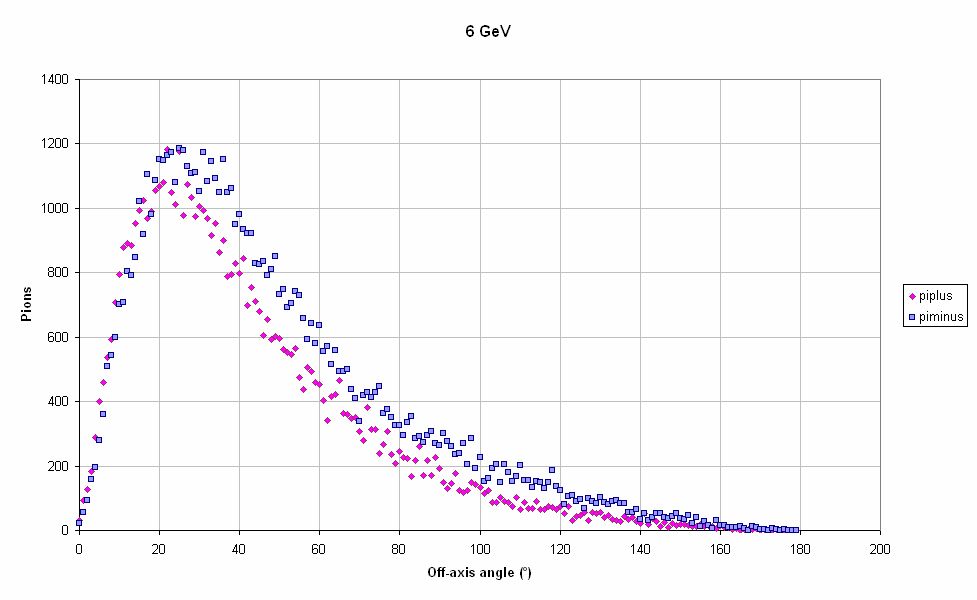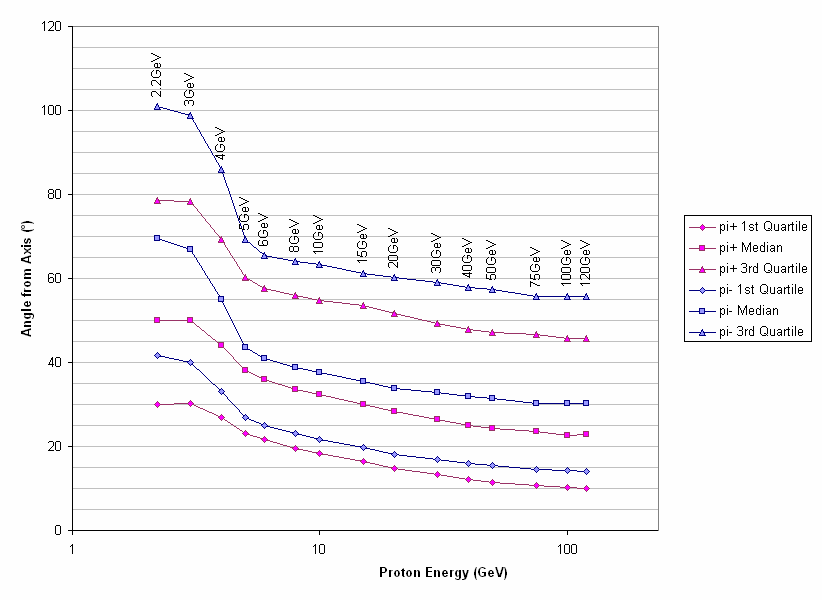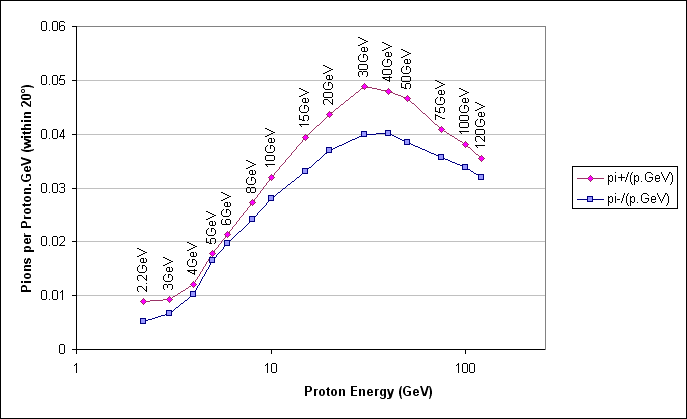# Simulations of Pion Production in a Tantalum Rod Target using MARS15

Stephen Brooks (s.j.brooks@rl.ac.uk)   January 2005
1.  Abstract

One way of producing an intense muon beam for a Neutrino Factory or Muon Collider is to collide protons with a target material to produce pions, which decay into muons.  This paper examines the case of a solid tantalum rod target and how the choice of proton energy affects the pion yield, both in total and in a forward directed cone useable in later capture optics.

2.  Simulation Setup

The newest revision of the MARS particle production code was used in order to get realistic results.  Unfortunately, data on pion production from the HARP experiment2 is not yet available, so has not yet been integrated into MARS.  When this happens, the simulations that follow will be rerun to see the impact of the new data.

 Table 1.  Primary simulation parameters.
Code Used MARS15(2004)1 105 Parallel beam, parabolically distributed on 1cm radius circle Tantalum Cylindrical 20cm length × 1cm radius

The proton beam hits the target end-on, parallel to the axis of the cylinder.  MARS was configured to record any π+ or π leaving through any of its surfaces.  See Appendix B for the input file used for these simulations.
It should be noted that a target of this length does not fully stop the proton beam, so some protons pass right through, albeit experiencing severe multiple scattering in the high Z material.  The target also produces copious quantities of neutrons; indeed, a stopping tantalum target is sometimes used in dedicated neutron production machines.

A radial parabolic distribution ρ(r)∝1−r2, 0≤r≤1, was used, with '1' scaled to the radius of the rod (see Appendix C for generating routine).  This is preferable to the rectangular and Gaussian beams that are defaults in MARS, as it is the same shape as the rod.  Another option frequently used in target studies is the uniform distribution on a circle, but this is hard to achieve in practice with a proton extraction line.  The parabolic distribution, however, is the projection into the plane of a 4D "waterbag" phase-space distribution, which is often used as a model of proton bunches.
The beam was assumed to be parallel for the purposes of the simulation, as typical proton drivers have beams with too small an angular divergence to greatly affect the results of a hadron shower calculation in a block of material.

3.  Proton Driver Energies

Results were generated for a selection of energies, listed in the table, including those for some candidate proton drivers already under study.

 Table 2.  Proton energies used in the simulations.
Proton Energy (GeV)Proposed Machines
2.2CERN SPL
3RAL ISIS upgrade low energy ring (primarily for neutrons)
4Possible highest energy for SPL
5RAL study for green field site
6RAL ISIS upgrade high energy ring [6-8GeV]
8FNAL driver study 2
10
15RAL study for machine the ISR tunnel at CERN; [FNAL driver study 1, 16GeV]
30JPARC high energy ring initial energy; also RAL study for a CERN PS replacement in the ISR tunnel
40
50JPARC high energy ring ultimate energy
75
100
120FNAL main injector energy, currently used for NuMI beam
4.  Pion Yield by Energy

The most obvious quantity to look at is the total number of pions leaving the rod for each proton energy.  The raw numbers are converted into pions per proton.GeV, which is proportional to the number of pions produced per watt of proton beam (pions per proton will always increase with energy).Figure 1.  Total pion yield per unit beam power.

For this figure, the dependence on energy is moderate, with the highest yield ~60% better than the worst.  The peak for π+ is at 30GeV and the peak for π is at 20GeV, though the graphs (figure 1) are roughly flat down to 15GeV.

5.  Pion Angular Distribution

The pions will be difficult to capture in a decay channel if they are travelling sideways or backwards from the rod.  Therefore it is important to measure the angular distribution for each energy, to make sure the high-yield energies are not producing an unusable beam.  Figures 2a-e plot the distribution of the angle (θ) between the emission velocity of the pions and the forward axis of the rod, in 1° bins.Figure 2a.  Pion angular distribution at 2.2GeV.Figure 2b.  Pion angular distribution at 6GeV.Figure 2c.  Pion angular distribution at 15GeV.Figure 2d.  Pion angular distribution at 50GeV.Figure 2e.  Pion angular distribution at 120GeV.

Note that the linear fall towards θ=0° is not a hole in the distribution but an effect of the element of area for each bin being 2π sin θ dθ.  One could divide the distribution by sin θ to see how the true density (per steradian) varies.

 Table 3.  Pion distributions for different energies, expressed as quartiles (25% fall in each of the ranges delimited by the three numbers and θ=0,180°).
Proton Energy (GeV)π+ Angle from Axis (°)π Angle from Axis (°)
1st QuartileMedian3rd Quartile 1st QuartileMedian3rd Quartile
2.230.0250.0278.5141.5869.47100.88
3 30.2250.0478.2840.0566.9798.80
4 26.8444.1169.3633.0554.9585.92
5 23.1938.1160.1526.9043.5669.23
6 21.5635.8557.7425.0341.0665.40
8 19.5233.4955.9122.9938.8164.06
10 18.2932.4454.6621.6737.6363.42
15 16.3930.0553.5219.6835.5861.28
20 14.8428.3251.7418.1533.8760.20
30 13.3726.5349.2016.7932.8158.94
40 12.1924.8847.8815.8831.8957.80
50 11.4724.3747.1815.4331.5357.38
75 10.6423.4946.6814.4330.1955.81
10010.1822.7345.7214.2230.1955.82
120 9.9122.7445.6814.0730.3055.67Figure 3.  Half-apex angles of cones that contain 25%, 50% and 75% of the pion distribution at various energies.

We see in figure 3 that the off-axis angle of the pions decreases with proton energy and the π distribution is always less focussed than that of the π+.

Unfortunately, the kink in the graph in the range 3–5GeV may not be physical, as the model that MARS uses for pion calculations changes over this range and the code mixes from one to the other.  The kink most likely indicates that the two models produce somewhat different results at around 4GeV.  Benchmarking of these results against those produced by the GEANT4 or FLUKA code for an identical setup is planned in the future.

6.  Pion Yield Within ±20° of Rod Axis

One way to combine the total pion yield and the angular distribution data into a single figure of merit is to count the pions emitted within a certain angle of the forward axis (i.e. with velocities inside a forward-pointing cone).  This is a fairly crude cut, designed to eliminate only pions whose direction is so far off-axis that they would fall outside the range of dynamics considered in any conventional decay channel.  The relatively large cutoff angle of 20° is therefore chosen.
For long-range acceptance into a regular focussing channel, transverse momentum might be a better figure to use, and this is done in the next section.Figure 4.  Yield of pions within 20° of the forward axis, per unit proton beam power.

Figure 4 shows a far steeper falloff at the low energy end than the graph of total pion yield, while the high energy decrease is shallower.  For this figure of merit, the lowest energy is 5.4× worse than the peak for π+ and 7.2× worse for π.  By contrast, the peaks are only respectively 38% and 25% better than the 120GeV value.
The best energy for π+ is still 30GeV but for π it has become 40GeV.  The upward skew is a consequence of the beam focussing with increasing proton momentum, an effect that is particularly important for π as they tend to be less focussed than the π+.

7.  Selected for Transverse Momentum Below 100MeV/c

While the analysis in the previous section is suitable for solid-angle type effects in the early few solenoids, a 20° spread of pions is harder to capture into a focussing channel if the pions have higher energies, since they will be less affected by the focussing system.  Here we additionally apply a transverse momentum threshold of 100MeV/c, which is roughly the transverse momentum transmitted by a decay channel studied earlier3.Figure 5.  Pions within 20° and with transverse momentum less than 100MeV/c, normalised to unit proton beam power.

Although some statistical noise is starting to appear in figure 5, it is clear enough to show that the momentum cut has reduced the very steep decrease at low energy, which is now only about a factor of two or three.  The noise makes it harder to identify a clear peak, though 30GeV still stands out as a good energy, with acceptable yields from 5GeV up.

8.  Conclusion

Pion distributions have been generated at a selection of energies in MARS15 for a simple but well-defined target model.  In all analyses, a 30GeV proton beam appears to produce a near-optimum pion yield, with a shallow dependence on either side so that halving or doubling this energy should not give a dramatic reduction in efficiency.  Proton driver energies below 5GeV appear consistently bad due to a high angular spread in the pion beam, with significant numbers emitted backwards.

The pion data sets themselves can be obtained by e-mailing the author.

9.  Future Work
• Confirmation/benchmarking of this paper's results using GEANT4.
• Addition of a 20T magnetic field to check if this has significant effect within the rod.
• Tracking of these distributions through various decay channel scenarios, to achieve realistic yield figures.
• Optimisation of decay channel systems3 for the suggested proton driver energy of 30GeV.
• Additional investigations of the target parameters, such as variation of rod radius.
A.  References
B.  MARS.INP Template File

The token '\$energy' in the file below is replaced by a number in GeV for each energy required.  MARS requires that this end in a decimal point if it is a whole number (for instance '6.' for 6GeV but '2.2' for 2.2GeV).  The token '\$hole' is always replaced by '0.' currently, as the rod is solid.

```Tantalum Rod Pion Production [TEMPLATE] (2005-Jan-06)
/home/csf/brooks/mars15/dat
C GUI switch
CTRL 0

C Generate 100000 events
NEVT 100000

C 0.01mm boundary precision, 2cm initial trial step
SMIN 0.001 2.

C Beam is protons(1), parallel gaussian(2) [now modified in BEG1 subroutine]
IPIB 1 2
C 0.5cm RMS transversely (so rod is 2sigma), 1ns RMS longitudinally
BEAM 0.5 0.5 5=29.9792458
C Energy in GeV
ENRG \$energy

C Two materials used: tantalum and vacuum
NMAT 2
MATR 'TA' 'VAC'

C Use 'R-sandwich' type geometry (default)
INDX 2=F
C Two R-sections: vacuum(#2) to \$hole cm radius, tantalum(#1) to 1cm radius, ending at z=20cm
NLTR 2
RSEC \$hole 1. 51=10 10 101=2 1
ZSEC 20. 1251=200

C We record particles at three surfaces, 1cm out, z=0-20cm; and r=0-1cm, z=0cm and z=20cm to fort.83 (81..90)
NSUR 3
RZTS 1. 1.  0. 20.  83.
0. 1.  0.  0.  83.
0. 1. 20. 20.  83.

STOP```
C.  Modification to User Subroutine 'BEG1' in m1504.f

This is the section of the long Fortran file 'm1504.f' that has been modified to generate the correct input proton distribution.

```*      PARAMETER (CLIGHT=29979245800.D0)
PARAMETER (PI=3.141592653589793238D+00)
C- - - - - - - - - - - - - - - - - - - - - - - - - - - -
C+++ INSERT YOUR SOURCE TERM HERE +++